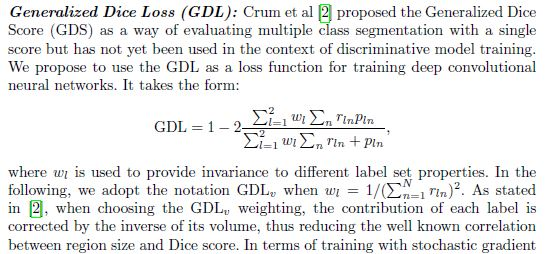# Generalized Dice Loss implementation issues

Hi there, so I’ve been working on implementing different loss functions for segmentation, partly by adapting work from:
https://github.com/LIVIAETS/surface-loss (work published: https://arxiv.org/abs/1812.07032)

Currently I’m just testing things in a colab notebook using the camvid example from part 1 to debug the adaptations.

Loss Function:

def GDL(input, target):
# -> Tensor: input = Batch x Classes x Pixels x Pixels, with Classes containing a probability map
for each class. target is classidx encoded

``````    #turn target into one_hot encoding and squeeze

target = class2one_hot(target) # Shape: Batch x Class(one-hot) x 1 x pixels x pixels
target = target.squeeze() # Shape: Batch x Class(one-hot) x pixels x pixels
assert one_hot(target) # asserts fine
#assert simplex(input)  #does not assert fine
assert simplex(target) #asserts fine

pc = input.type(torch.float32)
tc = target.type(torch.float32)

w = 1 / ((einsum("bcwh->bc", tc).type(torch.float32) + 1e-10) ** 2) #shape Batch x Classes
print('weight=',w.float().mean(),w.shape)

intersection = w * einsum("bcwh,bcwh->bc", pc, tc) #shape Batch x Classes
print('intersection=',intersection.float().mean(),intersection.shape)

union = w * (einsum("bcwh->bc", pc) + einsum("bcwh->bc", tc)) #shape Batch x Classes
print('union=',union.float().mean())

numerator = (einsum("bc->b", intersection) + 1e-10) #shape Batch x Classes
print('numerator=', numerator.float().mean(),intersection.shape)

denominator = (einsum("bc->b", union) + 1e-10) #shape Batch x Classes
print('denominator=', denominator.float().mean(),union.shape)

divided = 1 - 2 * (numerator / denominator) #Shape Batch
print('divided=',divided.float().mean(),divided.shape)
print('')

loss = divided.mean() #scalar

return loss
``````

Just to clarify, loss functions intake a Batch x Classes x Pixels x Pixels tensor, where classes are probability maps? in this case, target should indeed be one-hot encoded and same shape as input correct?

Loss function equation from paperExample Lr_find, finding some sort of lr-loss pattern but extremely small values?

Examples of output values and shapes during an epoch

I just feel like its not behaving as it should, producing extremely small numbers? in all cases, the numerator is very small and is divided by a very large number, resulting in 1-(very small number).

In the original code by the authors (in pytorch) (found here: https://github.com/LIVIAETS/surface-loss/blob/master/losses.py), they assert that input is simplex()=True, which does not work for me, so perhaps my input is different from theirs (custom training loop). The Simplex util is as follows:

def simplex(t, axis=1): #t: tensor-> bool:
_sum = t.sum(axis).type(torch.float32)
_ones = torch.ones_like(_sum, dtype=torch.float32)